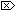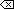The Open Group Base Specifications Issue 6
IEEE Std 1003.1-2001

#### NAME

atanh, atanhf, atanhl - inverse hyperbolic tangent functions

#### SYNOPSIS

```#include <math.h> double atanh(double x); float atanhf(float x); long double atanhl(long double x); ```

#### DESCRIPTION

[CX]The functionality described on this reference page is aligned with the ISO C standard. Any conflict between the requirements described here and the ISO C standard is unintentional. This volume of IEEE Std 1003.1-2001 defers to the ISO C standard.These functions shall compute the inverse hyperbolic tangent of their argument x.

An application wishing to check for error situations should set errno to zero and call feclearexcept(FE_ALL_EXCEPT) before calling these functions. On return, if errno is non-zero or fetestexcept(FE_INVALID | FE_DIVBYZERO | FE_OVERFLOW | FE_UNDERFLOW) is non-zero, an error has occurred.

#### RETURN VALUE

Upon successful completion, these functions shall return the inverse hyperbolic tangent of their argument.

If x is ±1, a pole error shall occur, and atanh(), atanhf(), and atanhl() shall return the value of the macro HUGE_VAL, HUGE_VALF, and HUGE_VALL, respectively, with the same sign as the correct value of the function.

For finite |x|>1, a domain error shall occur, and [MX]either a NaN (if supported), oran implementation-defined value shall be returned.

[MX]If x is NaN, a NaN shall be returned.

If x is ±0, x shall be returned.

If x is ±Inf, a domain error shall occur, and either a NaN (if supported), or an implementation-defined value shall be returned.

If x is subnormal, a range error may occur and x should be returned.#### ERRORS

These functions shall fail if:

Domain Error
The x argument is finite and not in the range [-1,1], [MX]or is ±Inf.If the integer expression (math_errhandling & MATH_ERRNO) is non-zero, then errno shall be set to [EDOM]. If the integer expression (math_errhandling & MATH_ERREXCEPT) is non-zero, then the invalid floating-point exception shall be raised.

Pole Error
The x argument is ±1.

If the integer expression (math_errhandling & MATH_ERRNO) is non-zero, then errno shall be set to [ERANGE]. If the integer expression (math_errhandling & MATH_ERREXCEPT) is non-zero, then the divide-by-zero floating-point exception shall be raised.

These functions may fail if:

Range Error
[MX]The value of x is subnormal.

If the integer expression (math_errhandling & MATH_ERRNO) is non-zero, then errno shall be set to [ERANGE]. If the integer expression (math_errhandling & MATH_ERREXCEPT) is non-zero, then the underflow floating-point exception shall be raised.The following sections are informative.

None.

#### APPLICATION USAGE

On error, the expressions (math_errhandling & MATH_ERRNO) and (math_errhandling & MATH_ERREXCEPT) are independent of each other, but at least one of them must be non-zero.

None.

#### FUTURE DIRECTIONS

None.

feclearexcept() , fetestexcept() , tanh() , the Base Definitions volume of IEEE Std 1003.1-2001, Section 4.18, Treatment of Error Conditions for Mathematical Functions, <math.h>

#### CHANGE HISTORY

First released in Issue 4, Version 2.

#### Issue 5

Moved from X/OPEN UNIX extension to BASE.

#### Issue 6

The atanh() function is no longer marked as an extension.

The atanhf() and atanhl() functions are added for alignment with the ISO/IEC 9899:1999 standard.

The DESCRIPTION, RETURN VALUE, ERRORS, and APPLICATION USAGE sections are revised to align with the ISO/IEC 9899:1999 standard.

IEC 60559:1989 standard floating-point extensions over the ISO/IEC 9899:1999 standard are marked.

End of informative text.

UNIX ® is a registered Trademark of The Open Group.
POSIX ® is a registered Trademark of The IEEE.
[ Main Index | XBD | XCU | XSH | XRAT ]